# Dynamics

## Concepts and Principles

Just like in kinematics, it’s an empirical fact about nature that when a force acts on an object in one direction (for example, the horizontal) this action does not appear to cause changes in the motion in a perpendicular direction (the vertical). Therefore, to investigate the effects of forces on the motion of an object in the vertical direction, you can ignore all forces acting in the horizontal direction. Of course, many forces will simultaneously act in both the horizontal and vertical directions. As in kinematics, the effect of these forces can be examined by concentrating on the components of the forces in the various directions. Again, as long as the directions of interest are perpendicular, the force components can be determined through right-angle trigonometry, and the magnitude of the force can always be determined by: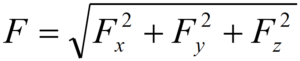Thus, Newton’s second law,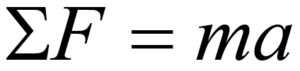is independently valid in any member of a set of perpendicular directions. The total force in the horizontal direction, for example, is equal to the mass times the acceleration in that direction. Note that the mass has been verified to be independent of direction, meaning that objects possess the same inertia in all directions.

## Analysis Tools

### Drawing Free-Body Diagrams

The free-body diagram is still the most important analysis tool for determining the forces that act on a particular object. As an example, start with a verbal description of a situation:

While rearranging furniture, a 600 N force is applied at an angle of 250 below horizontal to a 100 kg sofa at rest.

A free-body diagram for the sofa is sketched below: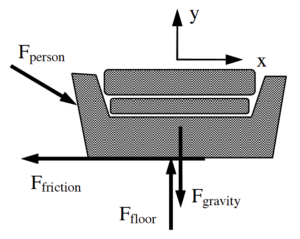The only non-contact interaction is the force of gravity, directed vertically downward. The couch is in contact with two external objects, the person, pushing the couch across the floor, and the floor, exerting a force directed upward to prevent the couch from sinking into the floor. In addition, experience tells us that the interaction between the couch and the floor also hinders the motion of the couch in the direction of the person’s push. This portion of the couch-floor interaction is commonly referred to as friction.

This is a complete free-body diagram for the couch.

### The Force of Friction

The interaction between objects in direct contact typically consists of two parts. One part of the interaction is directed perpendicular to the surface of contact. The other part of the interaction is the portion commonly called friction. The frictional portion of the interaction depends on many variables.

For most situations, a model of friction limiting the number of variables effecting the interaction to two is adequate. These two variables are the magnitude of the perpendicular portion of the interaction, generically called the contact force, and a unit-less constant that reflects the relative roughness of the surface-to-surface contact, termed the coefficient of friction. This linear model of sliding friction further differentiates between the frictional interaction when the two surfaces are moving with respect to each other, termed kinetic friction, and when they are not, termed static friction.

#### Kinetic friction

The kinetic friction model states that the frictional interaction between the surfaces is approximately equal to the product of the contact force, Fcontact and the coefficient of friction for kinetic situations, μk: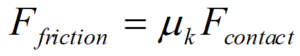The direction of this force on a particular object is in opposition to the relative motion of the two surfaces in contact.

#### Static friction

The static friction model states that the frictional interaction between the surfaces must be less than, or at most equal to, the product of the contact force, Fcontact, and the coefficient of friction for static situations, μs: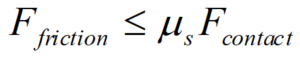The direction of this force on a particular object is in opposition to the motion that would result if the frictional interaction were not present.

### Applying Newton’s Second Law

Using this model for friction, we can now quantitatively analyze the original situation. Note that the two coefficients of friction will typically be given as an ordered pair, (ms, mk).

While rearranging furniture, a 600 N force is applied at an angle of 250 below horizontal to a 100 kg sofa at rest. The coefficient of friction between the sofa and the floor is (0.5, 0.4).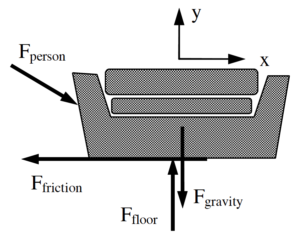Hopefully you realize that two quite different outcomes can result from this push. Either the person pushes too weakly to move the couch or the push is sufficient to make the couch move. Since the frictional forces acting in these two cases are quite different, we can’t really numerically analyze the situation until we make an assumption as to the outcome of the push. Of course, we will then have to check the validity of our assumption once we have completed our analysis. If our assumption turns out to be incorrect, we will then have to re-analyze the situation using the other possible outcome.

I will assume the couch doesn’t move for the analysis below, and then later check the assumption. Assuming the couch doesn’t move is equivalent to assuming ax = 0 m/s2 and that the relevant type of friction to use is static friction.

Applying Newton’s Second Law independently in the horizontal (x) and vertical (y) directions yields: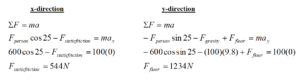Notice that the acceleration of the couch in the vertical direction must be zero regardless of my assumption, unless the couch begins to levitate or crash through the floor.

Assuming the couch doesn’t move leads to a calculated value of static friction equal to 544 N. Can static friction create a force of this magnitude to prevent the couch’s motion? I can check this calculated value against the allowed values for static friction: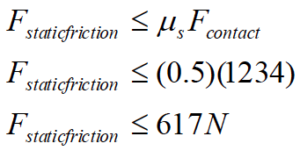Since the calculated value of the static frictional force is below the maximum possible value of the static frictional force, my analysis and assumption are valid, the couch does not budge. The person is not pushing hard enough to overcome the static frictional force that acts to prevent the couch’s motion relative to the floor.

Therefore, in this scenario the actual value of the static frictional force is 544 N (remember, it can be any value less than or equal to 617 N) and the acceleration of the couch is equal to zero.

How would the analysis change if the couch was initially in motion? Assume you enlisted a friend to help get the couch moving, but as soon as it began to move your friend stopped pushing. Would the couch stop immediately, gradually slow down to a stop, or could you keep the couch in motion across the room?

If the couch was initially moving, two things must change in our analysis. First, the horizontal acceleration of the couch is no longer necessarily zero. Second, the frictional force acting on the couch is kinetic.

Applying Newton’s Second Law independently in the horizontal (x) and vertical (y) directions now yields: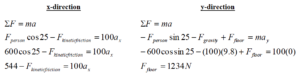To finish the analysis, we need to calculate the kinetic frictional force.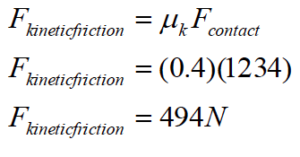Substituting this into the x-equation above yields: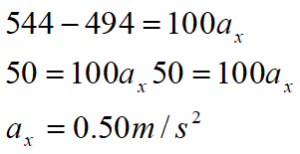Thus, if the couch is already moving the kinetic frictional force is 494 N and the couch accelerates toward the right at 0.50 m/s2. In summary, if the couch is initially moving it will continue to move and accelerate at 0.50 m/s2 to the right. If it is initially at rest, the person pushing on it will not be able to get it to move.

### Choosing a Coordinate System

In analyzing a scenario, you are always free to choose whatever coordinate system you like. If you make up negative, or left positive, this will not make you get the wrong answer. However, certain coordinate systems may make the mathematical analysis simpler than other coordinate systems. For example;

A 75 kg skier starts from rest at the top of a 200 slope. He’s a show-off, so he skies down the hill backward. The frictional coefficient between his skies and the snow is (0.10,0.05).

In attempting to analyze this situation, first draw a free-body diagram.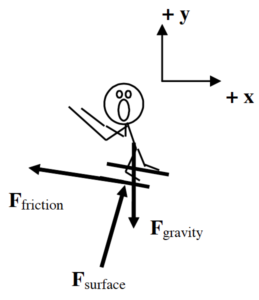Notice that I have chosen the traditional horizontal and vertical coordinate system. I could analyze the situation using this coordinate system, but there are two difficulties with this choice. 1.      Neither the force of the surface nor the force of friction is oriented in the x- or y-direction. (The force of gravity is oriented in the negative y-direction.) Therefore, I will have to use trigonometry to determine the x- and y-components of both of these forces. 2.      The skier is accelerating down the inclined slope. Thus, I will also need trigonometry to determine the x- and y-components of the acceleration.

Although these difficulties are by no means insurmountable, why make the task more difficult than it has to be?

Contrast the above choice of coordinate system with a coordinate system in which the x-direction is tilted parallel to the surface on which the skier slides and the y-direction, remaining perpendicular to the x, is perpendicular to the surface.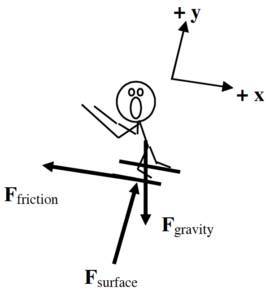1.      Using the tilted coordinate system, the only force not oriented in the x- or y-direction is the force of gravity. Therefore, I will only need to use trigonometry to determine the x- and y-components of one force rather than two. 2.      The skier is accelerating down the inclined slope. Since the x-direction is oriented parallel to the slope, the skier has an acceleration in the x-direction and zero acceleration in the y-direction.

This simple rotation of the coordinate system has made the mathematical analysis of this situation much easier. Applying Newton’s second law in the x- and y-direction leads to: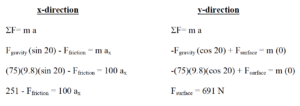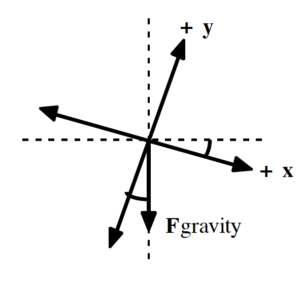Notice that if the x-axis is rotated by 200 from horizontal to become parallel to the slope, the y-axis is rotated by 200 from vertical. Since the force of gravity is always oriented vertically downward, it’s now 200 from the y-axis. Thus, the force of gravity has a component in the positive x-direction of Fgravity (sin 200) and a component in the negative y-direction of Fgravity (cos 200).

Now that the contact force between the skier and the slope is known, the static friction force can be determined.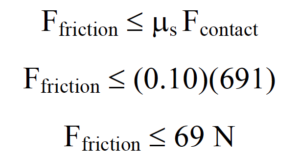Since the x-component of the force of gravity on the skier (251 N) is larger than the force of static friction (69 N), the skier will accelerate down the hill. Once he begins to move, the frictional force must be calculated using the kinetic friction model.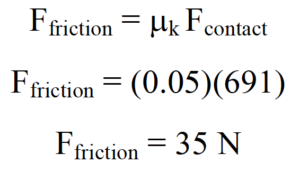Examining the x-component of Newton’s second law: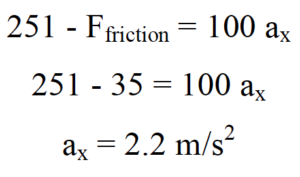The skier accelerates down the slope with an acceleration of 2.2 m/s2.

## Activities

Construct free-body diagrams for the objects described below.

Someone mistakenly put a lovely couch out at the curb on garbage day and you decide to take it back to your apartment. You push horizontally on the 80 kg couch with a force of 320 N. The frictional coefficient is (0.40,0.35).

 assuming the couch does not move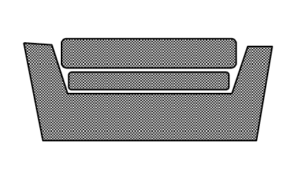assuming the couch does moveA 100 kg bicycle and rider initially move at 16 m/s up a 150 hill. The rider slams on the brakes and skids to rest. The coefficient of friction is (0.8,0.7).

 while skidding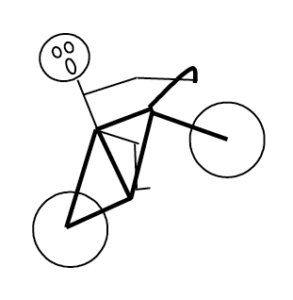when the bike is at rest on the inclineA 10 kg box is stacked on top of a 25 kg box. The boxes are at rest on an 8° incline.

 the top box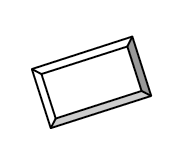the bottom box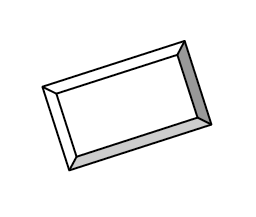Construct free-body diagrams for the objects described below.

Someone mistakenly put a lovely couch out at the curb on garbage day and you decide to take it back to your apartment. You pull on the 110 kg couch with a force of 410 N directed at 350 above horizontal. The frictional coefficient is (0.40,0.35).

 assuming the couch does not moveassuming the couch does moveA 60 kg skier starts from rest at the top of a 100 m, 250 slope. He doesn’t push with his poles because he’s afraid of going too fast. The frictional coefficient is (0.10,0.05).

 while skiing downhill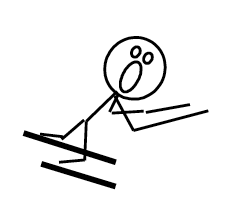while being pulled back uphill by the towrope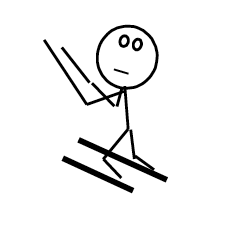A 10 kg box is stacked on top of a 25 kg box. The boxes are sliding down an 18° incline at increasing speed. The top box is not moving relative to the bottom box.

 the top boxthe bottom boxThe strange man below is trying to pull the pair of boxes up the incline. Construct the requested free-body diagrams.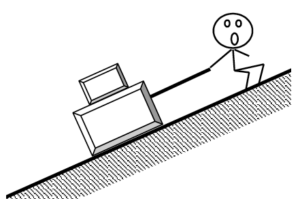The boxes almost move up the incline.

 the top boxthe bottom boxThe boxes move up the incline.

 the top boxthe bottom boxThe bottom box moves up the incline but the top box slides off the bottom box.

 the top boxthe bottom boxThe strange man below is trying to prevent himself from getting crushed by the boxes. Construct the requested free-body diagrams.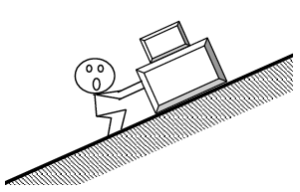The boxes almost move down the incline.

 the top boxthe bottom boxThe boxes almost move up the incline.

 the top boxthe bottom boxThe bottom box almost moves down the incline but the top box slides off the bottom box.

 the top boxthe bottom boxA constant magnitude force is applied to a rope attached to a crate. The crate is on a level surface. For each of the following situations, circle the correct relationship symbol between the two force magnitudes and explain your reasoning.

The crate moves at constant speed and the rope is horizontal.

 Fgravity >   =   <   ? Fsurface Frope >   =   <   ? Ffriction

Explanation:

The crate does not move and the rope is horizontal.

 Fgravity >   =   <   ? Fsurface Frope >   =   <   ? Ffriction

Explanation:

The crate moves at constant speed and the rope is inclined above the horizontal.

 Fgravity >   =   <   ? Fsurface Frope >   =   <   ? Ffriction

Explanation:

A constant magnitude force is applied to a rope attached to a crate. The crate is on an inclined surface. For each of the following situations, circle the correct relationship symbol between the two force magnitudes and explain your reasoning.

The crate does not move and the rope is parallel to the incline and directed up the incline.

 Fgravity >   =   <   ? Fsurface Frope >   =   <   ? Ffriction

Explanation:

The crate does not move and the rope is parallel to the incline and directed down the incline.

 Fgravity >   =   <   ? Fsurface Frope >   =   <   ? Ffriction

Explanation:

The crate moves at constant speed up the incline and the rope is parallel to the incline and directed up the incline.

 Fgravity >   =   <   ? Fsurface Frope >   =   <   ? Ffriction

Explanation:

Below are six crates at rest on level surfaces. The crates have different masses and the frictional coefficients between the crates and the surfaces differ. The same external force is applied to each crate, but none of the crates move. Rank the crates on the basis of the magnitude of the frictional force acting on them.

Largest  1. _____ 2. _____ 3. _____ 4. _____ 5. _____ 6. _____ Smallest

_____ The ranking cannot be determined based on the information provided.

Explain the reason for your ranking:

Below are six crates at rest on level surfaces. The masses, frictional coefficients between the crates and the surfaces, and the external applied force all differ.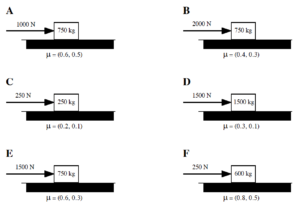If none of the crates move, rank the crates on the basis of the magnitude of the frictional force acting on them.

Largest  1. _____ 2. _____ 3. _____ 4. _____ 5. _____ 6. _____ Smallest

_____ The ranking cannot be determined based on the information provided.

Explain the reason for your ranking:

If the crates are moving, rank the crates on the basis of the magnitude of the frictional force acting on them.

Largest  1. _____ 2. _____ 3. _____ 4. _____ 5. _____ 6. _____ Smallest

_____ The ranking cannot be determined based on the information provided.

Explain the reason for your ranking:

Below are six boxes held at rest against a wall. The coefficients of friction between each box and the wall are identical.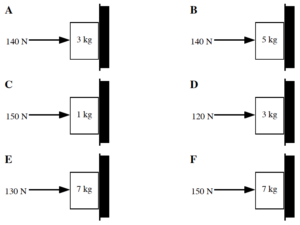Rank the boxes on the basis of the magnitude of the force of the wall acting on them.

Largest  1. _____ 2. _____ 3. _____ 4. _____ 5. _____ 6. _____ Smallest

_____ The ranking cannot be determined based on the information provided.

Explain the reason for your ranking:

Rank the boxes on the basis of the magnitude of the frictional force acting on them.

Largest  1. _____ 2. _____ 3. _____ 4. _____ 5. _____ 6. _____ Smallest

_____ The ranking cannot be determined based on the information provided.

Explain the reason for your ranking:

Below are eight crates of differing mass. Each crate is being pulled to the right at the same constant speed.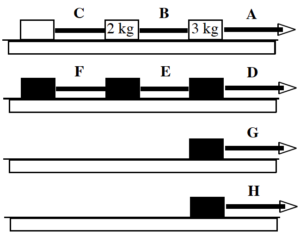Rank the magnitude of the force exerted by each rope on the crate immediately to its left if the frictional coefficient between each crate and the surface is the same non-zero value.

Largest  1. _____ 2. _____ 3. _____ 4. _____ 5. _____ 6. _____ Smallest

_____ The ranking cannot be determined based on the information provided.

Explain the reason for your ranking:

Rank the magnitude of the force exerted by each rope on the crate immediately to its left if the frictional coefficient between each crate and the surface is zero.

Largest  1. _____ 2. _____ 3. _____ 4. _____ 5. _____ 6. _____ Smallest

_____ The ranking cannot be determined based on the information provided.

Explain the reason for your ranking:

Below are eight crates of differing mass. The frictional coefficients between each crate and the surface on which they slide are so small that the force of friction is negligible on all crates. Each crate is being pulled to the right and accelerating. The acceleration of each crate or chain of crates is given. Rank the magnitude of the force exerted by each rope on the crate immediately to its left.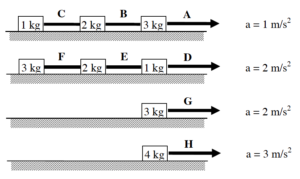Largest 1. _____ 2. _____ 3. _____ 4. _____ 5. _____ 6. _____7. _____ 8. _____Smallest

_____ The ranking cannot be determined based on the information provided.

Explain the reason for your ranking:

Someone mistakenly put a lovely couch out at the curb on garbage day and you decide to take it back to your apartment. You push on the 110 kg couch with a force of 410 N directed at 350 below horizontal. The couch doesn’t move.

Free-Body Diagram

Mathematical Analysis[i]

x-direction                                                                                                                                  y-direction

Someone mistakenly put a lovely couch out at the curb on garbage day and you decide to take it back to your apartment. You push on the 80 kg couch with a force of 320 N directed at 150 below horizontal. The frictional coefficient is (0.40,0.35).

Free-Body Diagram

Mathematical Analysis[ii]

x-direction                                                                                                                                  y-direction

Someone mistakenly put a lovely couch out at the curb on garbage day and you decide to take it back to your apartment. You pull on the 110 kg couch with a force of 510 N directed at 350 above horizontal. The frictional coefficient is (0.40,0.35).

Free-Body Diagram

Mathematical Analysis[iii]

x-direction                                                                                                                                  y-direction

You get into a fight with another person over a garbage-day couch. You push on the 80 kg couch with a force of 660 N directed at 150 below horizontal. She claims ownership by sitting on the couch while you try to push it. You still manage to just barely get the couch moving. The frictional coefficient is (0.40,0.35).

Free-Body Diagram

Mathematical Analysis[iv]

x-direction                                                                                                                                  y-direction

You get into a fight with another person over a garbage-day couch. You push on the 80 kg couch with a force of 420 N directed at 150 below horizontal. She pushes on the other side of the couch with a force of 510 N directed at 250 below horizontal. The frictional coefficient is (0.40,0.35).

Free-Body Diagram

Mathematical Analysis[v]

x-direction                                                                                                                                  y-direction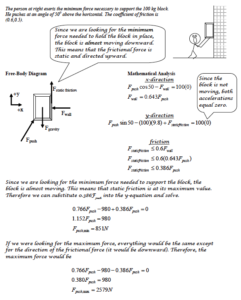The person at right exerts an 850 N force on the 90 kg block at an angle of 550 above the horizontal. The coefficient of friction is (0.6,0.5).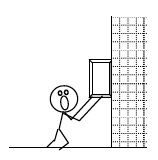Free-Body Diagram

Mathematical Analysis[vi]

x-direction                                                                                                                                  y-direction

 The person at right exerts a 620 N force on the 70 kg block at an angle of 400 above the horizontal. The coefficient of friction is (0.5,0.4).Free-Body Diagram

Mathematical Analysis[vii]

x-direction                                                                                                                                  y-direction

A boy pulls a 30 kg sled, including the mass of his kid brother, along ice. The boy pulls on the tow rope, oriented at 600 above horizontal, with a force of 110 N until his kid brother begins to cry. Like clockwork, his brother always cries upon reaching a speed of 2.0 m/s. The frictional coefficient is (0.20,0.15).

Motion Information                                                                   Free-Body Diagram

 Event 1:   t1 = r1x = r1y = v1x = v1y = a12x = a12y = Event 2:   t2 = r2x = r2y = v2x = v2y =

Mathematical Analysis[viii]

x-direction                                                                                                                                  y-direction

Starting from rest, a girl can pull a  sled, carrying her kid brother, 20 m in 8 s. The girl pulls on the tow rope, oriented at 300 above horizontal, with a force of 90 N. The frictional coefficient is (0.15,0.10).

Motion Information                                                                   Free-Body Diagram

 Event 1:   t1 = r1x = r1y = v1x = v1y = a12x = a12y = Event 2:   t2 = r2x = r2y = v2x = v2y =

Mathematical Analysis[ix]

x-direction                                                                                                                                  y-direction

A 60 kg skier starts from rest at the top of a 100 m, 250 slope. He doesn’t push with his poles because he’s afraid of going too fast. The frictional coefficient is (0.10,0.05).

Motion Information                                                                   Free-Body Diagram

 Event 1:   t1 = r1x = r1y = v1x = v1y = a12x = a12y = Event 2:   t2 = r2x = r2y = v2x = v2y =

Mathematical Analysis[x]

x-direction                                                                                                                                  y-direction

A 100 kg bicycle and rider initially move at 16 m/s up a 150 hill. The rider slams on the brakes and skids to rest. The coefficient of friction is (0.8,0.7).

Motion Information                                                                   Free-Body Diagram

 Event 1:   t1 = r1x = r1y = v1x = v1y = a12x = a12y = Event 2:   t2 = r2x = r2y = v2x = v2y =

Mathematical Analysis[xi]

x-direction                                                                                                                                  y-direction

A 70 kg snowboarder starts from rest at the top of a 270 m, 200 slope. She reaches the bottom of the slope in 14.5 seconds.

Motion Information                                                                   Free-Body Diagram

 Event 1:   t1 = r1x = r1y = v1x = v1y = a12x = a12y = Event 2:   t2 = r2x = r2y = v2x = v2y =

Mathematical Analysis[xii]

x-direction                                                                                                                                  y-direction

At a UPS distribution center, a 60 kg crate is at rest on an 8° ramp. A worker applies the minimum horizontal force needed to push the crate up the ramp. The coefficient of friction between the crate and the ramp is (0.3, 0.2).

Free-Body Diagram

Mathematical Analysis[xiii]

x-direction                                                                                                                                  y-direction

At a UPS distribution center, a 40 kg crate is sliding down an 8° ramp at 3 m/s. A worker applies a horizontal force to the crate and brings the crate to rest in 1.5 s. The coefficient of friction between the crate and the ramp is (0.3, 0.2).

Motion Information                                                                   Free-Body Diagram

 Event 1:   t1 = r1x = r1y = v1x = v1y = a12x = a12y = Event 2:   t2 = r2x = r2y = v2x = v2y =

Mathematical Analysis[xiv]

x-direction                                                                                                                                  y-direction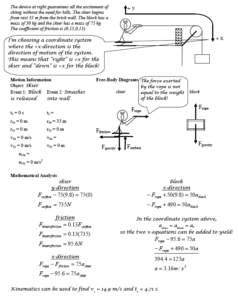The device at right allows novices to ski downhill at reduced speeds. The block has a mass of 15 kg and the skier has a mass of 70 kg. The coefficient of friction is (0.05,0.04). The skier starts from rest at the top of a 30 m, 200 slope.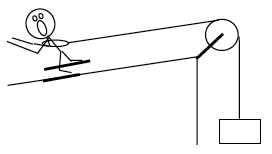Motion Information                                                       Free-Body Diagrams

Object:

 Event 1:   t1 = r1x = r1y = v1x = v1y = a12x = a12y = Event 2:   t2 = r2x = r2y = v2x = v2y = skier block

Mathematical Analysis[xv]

 The device at right allows you to ski uphill. The ballast block has a mass of 30 kg and the skier has a mass of 60 kg. The coefficient of friction is (0.07,0.06). The skier starts from rest at the bottom of a 30 m, 200 slope.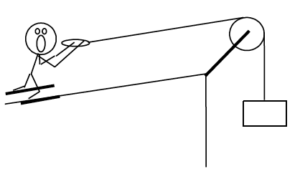Motion Information                                                       Free-Body Diagrams

Object:

 Event 1:   t1 = r1x = r1y = v1x = v1y = a12x = a12y = Event 2:   t2 = r2x = r2y = v2x = v2y = skier block

Mathematical Analysis[xvi]

 The device at right may allow you to ski uphill (or it may allow you to ski downhill backward). The ballast block has a mass of 20 kg and the skier has a mass of 70 kg. The coefficient of friction is (0.1,0.09). The ramp is inclined at 200 above horizontal.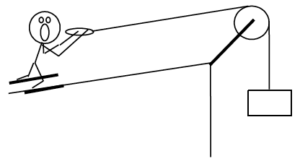Free-Body Diagrams

 skier block

Mathematical Analysis[xvii]

 The strange man at right wants to pull the two blocks to the other side of the room in as short a time as possible. However, he doesn’t want the top block to slide relative to the bottom block. The coefficient of friction between the bottom block and the floor is (0.25,0.20) and the coefficient of friction between the top block and the bottom block is (0.30,0.25). The blocks start from rest.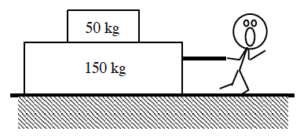Free-Body Diagrams

 top block bottom block

Mathematical Analysis[xviii]

 The strange man at right wants to pull the two blocks to the other side of the room in as short a time as possible by pulling on the top block. However, he doesn’t want the top block to slide relative to the bottom block. The coefficient of friction between the bottom block and the floor is (0.10,0.05) and the coefficient of friction between the top block and the bottom block is (0.60,0.50). The blocks start from rest.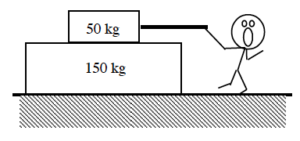Free-Body Diagrams

 top block bottom block

Mathematical Analysis[xix]

 The strange man at right wants to pull the two blocks to the top of the hill in as short a time as possible. However, he doesn’t want the top block to slide relative to the bottom block. The coefficient of friction between the 150 kg bottom block and the floor is (0.25,0.20) and the coefficient of friction between the 50 kg top block and the bottom block is (0.30,0.25). The hill is inclined at 150 above horizontal. The blocks start from rest.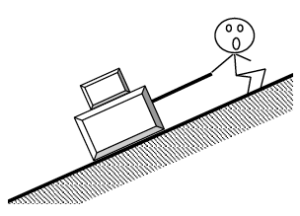Free-Body Diagrams

 top block bottom block

Mathematical Analysis[xx]

 The strange man at right applies the minimum force necessary to not get crushed by the bottom block. (The top block may or may not crush him.) The coefficient of friction between the 150 kg bottom block and the floor is (0.25,0.20) and the coefficient of friction between the 50 kg top block and the bottom block is (0.40,0.35). The hill is inclined at 200 above horizontal. The blocks are initially at rest.Free-Body Diagrams

 top block bottom block

Mathematical Analysis[xxi]

You should know the story by now. You push on a garbage-day couch at an angle q below horizontal. Determine the minimum force (Fmin) needed to move the couch as a function of  the couch’s mass (m), q, the appropriate coefficient of friction, and g.

Free-Body Diagram                                                                                                                    Mathematical Analysis

Questions

If q = 0°, what should Fmin equal? Does your function agree with this observation?

If q = 90°, what should Fmin equal? Does your function agree with this observation?

If m = ∞, what should Fmin equal? Does your function agree with this observation?

 The man at right exerts a force on the block at an angle q above horizontal. Determine the minimum force (Fmin) needed to begin to slide the block up the wall as a function of  the block’s mass (m), q, the appropriate coefficient of friction, and g.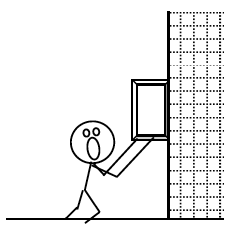Free-Body Diagram                                                                                                                    Mathematical Analysis

Questions

If q = 90°, what should Fmin equal? Does your function agree with this observation?

If q = 0°, what should Fmin equal? Does your function agree with this observation?

Below what angle q is it impossible to slide the block up the wall?

A crate is held at rest on a ramp inclined at q from horizontal. Determine the minimum force (Fmin), applied parallel to the incline, needed to prevent the crate from sliding down the ramp as a function of  the crate’s mass (m), q, the appropriate coefficient of friction, and g.

Free-Body Diagram                                                                                                                    Mathematical Analysis

Questions

If q = 0°, what should Fmin equal? Does your function agree with this observation?

If q = 90°, what should Fmin equal? Does your function agree with this observation?

If m = ∞, what should Fmin equal? Does your function agree with this observation?

A crate is held at rest on a ramp inclined at q from horizontal. Determine the maximum force (Fmax), applied horizontally, before the crate begins to move as a function of  the crate’s mass (m), q, the appropriate coefficient of friction, and g.

Free-Body Diagram                                                                                                                    Mathematical Analysis

Questions

If m = ∞, what should Fmax equal? Does your function agree with this observation?

If g = ∞, what should Fmin equal? Does your function agree with this observation?

If q = 0°, what should Fmax equal? Does your function agree with this observation?

A skier of mass m starts from rest at the top of a ski run of incline q. Determine the minimum angle (qmin) such that the skier will begin to slide down the slope without pushing off as a function of m, the appropriate coefficient of friction, and g.

Free-Body Diagram                                                                                                                    Mathematical Analysis

Questions

If m = 0, what should qmin equal? Does your function agree with this observation?

If g = 0 m/s2, what should qmin equal? Does your function agree with this observation?

If m was twice as large, what should qmin equal? Does your function agree with this observation?

 The portion of the interaction directed perpendicular to the surface of contact is sometimes referred to as the normal force, where normal has its mathematical definition of perpendicular.

[i] ms ≥ 0.256

[ii] a = 0 m/s2

[iii] a = 0.94 m/s2

[iv] m = 65.2 kg

[v] Fsf = 56 N

[vi] Fsf = 186 N up

[vii] a = 1.39 m/s2 down

[viii] a = 0.84 m/s2

[ix] m = 51.4 kg

[x] t2 = 7.4 s

[xi] r2x = 14 m

[xii] mk = 0.085

[xiii] F = 270 N

[xiv] F = 55.9 N

[xv] a = 0.73 m/s2

[xvi] a = 0.66 m/s2

[xvii] a = 0 m/s2

[xviii] Fmax = 980 N

[xix] Fmax = 359 N

[xx] Fmax = 947 N

[xxi] Fmin = 210 N

Homework 5 – Model 2: 64, 68, 70, 71, 73, 77, 85, 92, 95, and 101.

1. The portion of the interaction directed perpendicular to the surface of contact is sometimes referred to as the normal force, where normal has its mathematical definition of perpendicular.